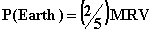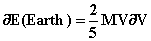Frequently Asked Questions

Series #10
Relativity and the GPS

( Last checked 2017/01/15 - The estate of Paul Marmet )
Goto: Next series of questions
Previous series of questions
List of papers on the Web
Goto Previous Question
--------------- ------------- ----------------- --------------
Comments by P. Marmet on the paper:
------------------------------------------------
Clock Behavior and the Search for an Underlying Mechanism
for Relativity Phenomena.
by Ronald R. Hatch
Presented by Ronald R. Hatch at an ION Meeting.
-----------------------------------------------
These comments demonstrate that it is incorrect to assume that a clock moving from the Earth pole to the equator is equivalent to an orbiting clock passing from perigee to apogee.  In the above paper, R. Hatch recalls that observations with the Global Positioning System GPS, (and other accurate experiments) show that an atomic clock maintains exactly the same rate when it is carried from the Earth pole at sea level, to the equator, also at sea level.  The Earth polar radius is smaller than the equator radius. Therefore, it requires energy to raise a mass higher, between the pole and the equator.  Furthermore, at the same time, due to the Earth rotation, at the equator that mass becomes accelerated to the velocity of the equator, which is 1670 Km/hr.  In the same paper, it is also recalled that when a satellite moves in an elliptical orbit around the Earth, the clock rate is slower when the satellite is closer to Earth (perigee) than when it is at maximum distance from Earth (apogee).  Of course, it is well known that the gravitational potential increases when the distance increases above the Earth, and that the velocity (kinetic energy) of the satellite increases when the satellite gets closer (perigee) to the Earth.
R. Hatch expresses his surprise to discover that there is an increase of clock rate when the satellite is at apogee, while there is no change of clock rate when the other clock experiment moves from the pole to a larger Earth radius at the equator.  Consequently, he claims that this requires a “new underlying relativistic phenomenon”.   Using Newton’s laws, we show here that this is a natural phenomenon.
Let us consider first the orbiting satellite.  When it passes from the perigee to the apogee, its orbiting velocity decreases (decrease of kinetic energy), and its potential energy increases by the same amount.  Therefore, the total energy of the satellite where the clock is located remains the same, everywhere on the orbit.
It is well known that a clock runs faster when there is an increase of potential energy.  Also, a clock runs faster when there is a decrease of velocity (kinetic energy).  These two mechanisms have been explained naturally and independently using mass-energy conservation and quantum mechanics. (Ref. 1 and Ref. 2).  Consequently, there is a double increase of clock rate when a clock travels from perigee and apogee as observed experimentally.  A similar situation explains the advance of the perihelion of Mercury. (Ref 3).
However, when we examine a clock carried from the Earth pole to the equator, we must realize that the problem is quite different.  Contrary to the example of the orbiting satellite, there is then no conservation of energy within the moving clock frame.  We need to add potential energy to raise the clock from the pole to the equator, plus kinetic energy due to the velocity of rotation of the Earth.  The sum of those two energies is twice the kinetic energy at the equator.
Consequently, that clock increases its rate due to the addition of potential energy, but it is slowed down due to the increase of velocity (kinetic energy) as explained above.  Therefore it is normal that there is no change of clock rate when the clock moves from the stationary Earth pole to the rotating equator, since the two phenomena are compensating the clock rate.  Contrary to Hatch suggestion, no new underlying relativistic mechanism is required.  Everything is normal when we apply the principle of mass-energy conservation and the mathematics of quantum mechanics (Ref 1, 2, 3 and 4).

Source of Energy Between the Pole and the Equator.
Let us examine how the principle of energy conservation is applied to the two experiments above.  In the case of the orbiting satellite, it is well known that the total energy of the satellite is conserved because the sum of potential plus kinetic energy everywhere along the orbit is equal to zero.  There is no exchange of energy between the orbiting satellite and the Earth.
However, we have seen above that when a mass moves from the pole to the rotating equator, there is a net increase of energy (potential plus kinetic), given to that moving clock.  Since it requires no external energy to carry a mass slowly between the Earth pole and the equator (neglecting friction), some people believe that there is no difference of energy.  That is an error, as we will see now.   Let us calculate where that energy comes from.
When a mass is at the Earth pole, its angular momentum around the Earth axis of rotation is zero.  Let us slowly move the clock away from the Earth pole.  When that clock, moving along a meridian, gets to an increasing distance from the pole, the rotating Earth makes it move faster and faster in a direction perpendicular to the meridian.  It is the inertia of the rotating Earth, which accelerates the moving clock in a transverse direction, until the clock reaches the equator and attains the transverse velocity of the equator, which is 1670 Km/hr.
At the same time the clock moves away from the Earth pole, another phenomenon takes place.  Since the equator is further away from the Earth center, a radial force is required to maintain the effortless motion of the clock along the meridian, which becomes larger near the equator.  That effortless motion is natural, since the Earth rotation produces a centrifugal force away from the pole in the direction of the equator. Those two forces compensates naturally.  In fact, the exact shape of the Earth is the natural equilibrium between these forces.
Let us calculate first the total increase of energy DE(clock) given to the clock moving from the pole to the equator.  It is equal to the potential energy plus the kinetic energy.
DE(clock) = (kinetic energy) + (potential energy)               1
Taking into account the flattened shape of the Earth and the centrifugal force, it is well known that the potential energy is equal to the kinetic energy.  Therefore the total increase of energy of the clock is:
DE(clock) =2 (kinetic energy)                                                2
The kinetic energy of the clock at the equator is
K.E. (clock)=½mV2                                                                  3
Where m is the mass of the clock and V is the velocity of the Earth equator.
Equation 3 in 2 gives that the total increase of energy DE(clock) of the clock moving from the Earth pole to the equator.
DE(clock) =2 (½mV2)=mV2.                                                4
The clock at Earth pole has zero angular momentum “P” with respect to the Earth axis (because R=0).  At the equator, the increase of angular momentum DP of the clock around the Earth axis is:
DP(clock)=mVR                                                                    5
Where R is the equatorial radius of the Earth.
We know that the Earth is an inertial frame in space and its total angular momentum remains constant even when a mass (like a clock) moves on its surface.  Since there is no external force acting on the Earth, its total angular momentum is constant.  We know that the moment of inertia “I” of a spheroid of revolution (as the Earth) along the polar axis is6
We have M is the mass of the Earth.  The angular momentum “P” around its axis is:
P=Iw                                                                                       7
The angular velocity w is defined as:8
Using equations 6, 7 and 8, we find that the total Earth angular momentum “P” before the clock moves to the equator is:9
When the clock is initially at the Earth pole, its mass brings no contribution to the Earth angular momentum (R=0). However, after the mass of the clock reaches the equator, its mass gives a new contribution to the angular momentum.  However, since Newton’s laws requires that the total Earth angular momentum is constant, the new angular momentum of the clock at the equator appears at the detriment of the total initial Earth angular momentum.
Equation 5 gives the change of angular momentum due to the displacement of the clock.  The relative change of angular momentum of the Earth (minus the clock) due to the displacement of the clock is obtained using equations 5 and 9.  This gives:10
Taking the derivative of equation 9 for the same Earth mass M at the same distance R (equator) we find:11
Equation 11 in 10 gives12
Let us calculate the energy corresponding to that change of Earth velocity.  The energy of a rotating body is
E(Earth)=½ Iw2                                                      13
Equations 6 and 7 in 13 give:14
The variation of the Earth rotational energy is given by the derivative of equation 14.  This gives:15
Using equation 15 let us calculate the total loss of the Earth rotational energy due to the displacement of the clock from the pole to the equator.  Let us substitute equation 12 in 15.  This gives:16
We find that equation 16 is identical to equation 4.  Therefore the energy acquired by the clock at the equator is equal to the energy lost due to the slowing down of the Earth rotation.  Therefore,17
Equation 17 shows that the increase of energy of the clock moving from the pole to the equator is exactly equal to the lost of kinetic energy of the rotating Earth.  Therefore, as expected, supplementary energy is really needed to move the clock from the pole to the equator.  However, that energy comes from the kinetic energy of the rotating Earth.  Therefore there is mass-energy conservation and also angular momentum conservation in the Earth frame.  Therefore is no need to use any force to carry the clock from the pole to the equator, because the internal Earth energy of rotation transfers that energy naturally.
The whole Earth is slowed down when a mass is moved from the pole to the equator as demonstrated here.  For that reason, the height of the sea level is in a natural equilibrium, higher at the equator than at the pole.  Unfortunately, Hatch paper neglects the fact that the mass of the clock has absorbed energy when passing from the pole to the equator while there is no change of energy between perigee and apogee.  We have seen that the increase of kinetic energy slows down the clock at the same time as an increase of potential energy speeds it up.  This explains the fact that the clock rate does not change when we move on the Earth surface remaining at sea level.  No new theory is required.  Conversely, in the case of an orbiting clock, there is no change of total energy between the perigee and apogee so that this leads naturally to a change of clock rate between perigee and apogee of an orbiting body.  Those two completely different experiments must not be confused.

References
1- Natural Length Contraction Mechanism Due to Kinetic Energy.  On the web at:
newtonphysics.on.ca/kinetic/index.html
2- Natural Physical Length Contraction Due to Gravity. On the Web at:
newtonphysics.on.ca/gravity/index.html
3 - A Detailed Classical Description of the Advance of the Perihelion of Mercury. On the web at:
newtonphysics.on.ca/mercury/index.html
4 – Einstein’s Theory of Relativity Versus Classical Mechanics
P. Marmet, Book, (1997) Ed. Newton Physics Books, Ogilvie Rd. Ottawa, Canada, K1J 7N4
On the Web at:
newtonphysics.on.ca/einstein/index.html
---------------------------------------
Paul Marmet, April 4, 2002

<><><><><><><><><><><><>
New Choice of Questions

<><><><><><><><><><><><><>

Goto : Next series of questions